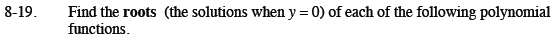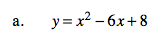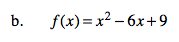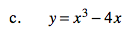Home > CCA2 > Chapter 8 > Lesson 8.1.1 > Problem8-19

8-19.
1. Find the roots (the solutions when y = 0) of each of the following polynomial functions. Homework Help ✎

1. y = x2 − 6x + 8

2. f(x) = x2 − 6x + 9

3. y = x3 − 4xFactor the right side and use the Zero Product Property.

x = 2, x = 4The roots are the x-intercepts on the graph of the function.

x = 3x is a common factor.

See part (a).